## Excel IPMT Function

The Excel IPMT function to figure out the interest payment of a given period in an investment or loan, based on periodic, constant payments and constant interest rate in Excel.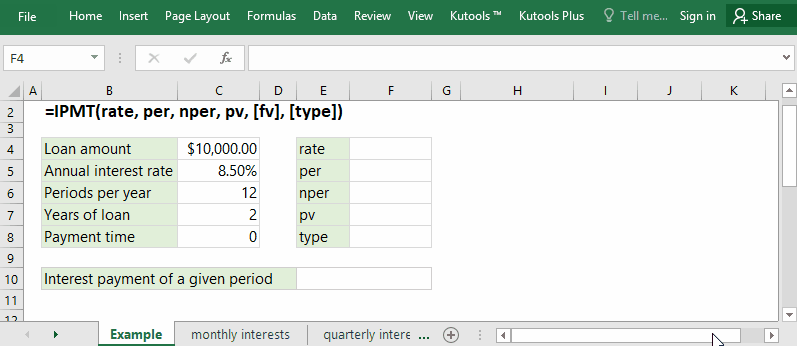#### Function syntax and arguments

IPMT(rate, per, nper, pv, [fv], [type])

(1) Rate: Required. It’s the interest rate per period.

The rate must be consistent with payment periods. If the annual interest rate is 6%, you can change it as follows:

• For semi-annual payments, you need to convert it to semi-annual interest rate: 3% (=6%/2);
• For quarterly payments, you need to convert it to quarterly interest rate: 1.5% (=6%/4);
• For monthly payments, you need to convert it to monthly interest rate: 0.5% (=6%/12).

(2) Per: Required. It’s the specified period that you will figure out the interest payment for. It’s must be in the range 1 to Nper.

(3) Nper: Required. It’s the total number of payment periods.

Most of time, you can get the information of the life of an investment or loan, and the payment frequency. For example, there is a 3-year investment, and you can figure out the total number of payment periods as follows:

• For semi-annual payments, the total number of periods is 6 (=3*2);
• For quarterly payments, the total number of periods is 12 (=3*4);
• For monthly payments, the total number of periods is 36 (=3*12).

(4) Pv: Required. It’s the present value, or the lump-sum amount that a series of future payments are worth at present.

(5) Fv: Optional. It’s the future value, or a cash balance you want to attain after the last payment is made. If omitted, it will be taken as 0.

(6) Type: Optional. A value indicates the time of payment. There are two types:

• 0 or omitted: payment at the end of every period;
• 1: payment at the beginning of every period.

#### Return Value

Numerical value.

The IPMT function returns the interest payment for the specified period for an investment or load, based on periodic, constant payments and a fixed interest rate.

#### Usage notes

(1) The rate must be consistent with payment periods.

(2) For cash out, the pv value and fv value should be negative numbers; for cash received, the fv value and pv value should be positive.

(3 The IPMT function returns #NUM! error value, if the nper value is less than 0.

(4) The IPMT function returns #NUM! error value, if the per value is less than 1 or greater than nper value.

(5) The IPMT function returns #VALUE! error value, if any of the supplied argument are non-numeric.

#### Formula Examples

##### Example 1: Calculate monthly interest payments on a credit card in Excel

For example, you sign a credit card installment agreement, and you will pay your bill of \$2,000 in 12 months with annual interest rate of 9.6%. In this example, you can apply the IPMT function to calculate the interest payment per month easily. Click to read more...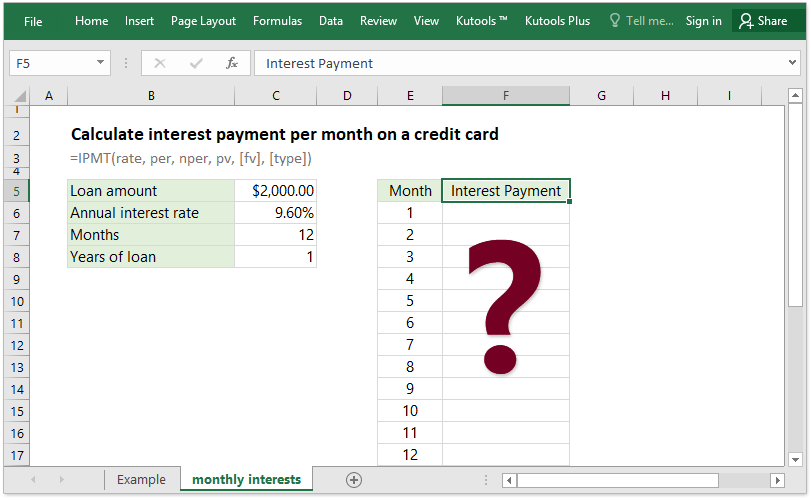##### Example 2: Calculate quarterly interest payments for a loan in Excel

Supposing you have a loan of \$10,000 from your bank, and the loan rate is 8.5%. From now on you need to pay back the loan in quarterly installment in 2 years. Now you can also apply the IPMT function to calculate the interest payment per quarter easily in Excel. Click to read more...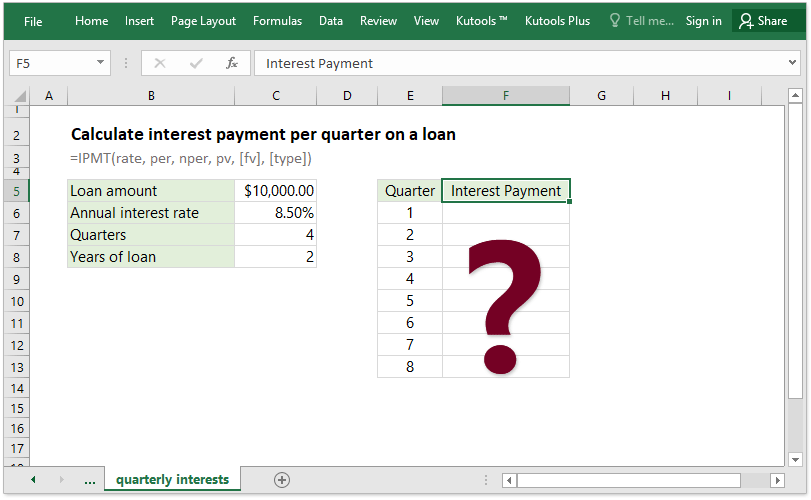##### Example 3: Calculate semi-annual interest payments on a car loan in Excel

Assume you are going to buy a car with a bank loan. The amount of this bank loan is \$50,000, its interest rate is 6.4%, you will repay the loan twice at the end of every half year, and the life of this bank loan is 5 years. In this case, you can easily figure out how much interest you will pay semi-annually easily with the IPMT function in Excel. Click to read more...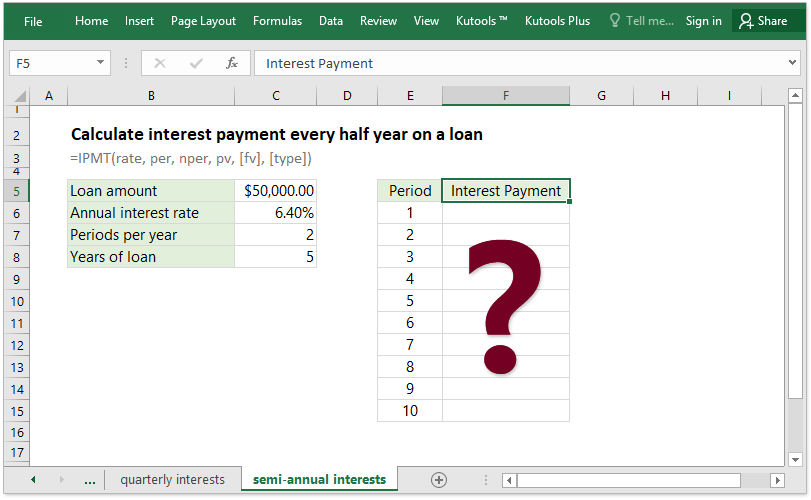##### Example 4: Calculate total interest paid on a loan in Excel

Sometimes, you may want to calculate the total interest paid on a loan. For periodic, constant payments and constant interest rate, you can apply the IPMT function to figure out the interest payment for every period, and then apply the Sum function to sum up these interest payments, or apply the CUMIPMT function to get the total interest paid on a loan directly in Excel.
For example, you have a loan of \$5,000 with annual interest rate of 8.00%. Now you need to repay it monthly in half year. Click to read how to figure out the total interest paid.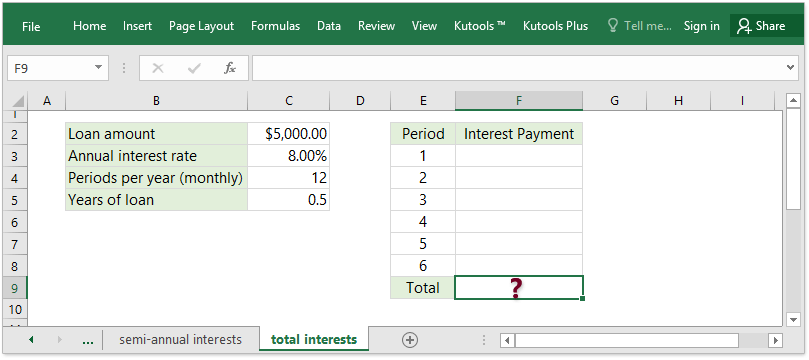### The Best Office Productivity Tools

#### Kutools for Excel - Helps You To Stand Out From Crowd

 Popular Features: Find, Highlight or Identify Duplicates  |  Delete Blank Rows  |  Combine Columns or Cells without Losing Data  |  Round without Formula ... Super VLookup: Multiple Criteria  |  Multiple Value  |  Across Multi-Sheets  |  Fuzzy Lookup... Adv. Drop-down List: Easy Drop Down List  |  Dependent Drop Down List  |  Multi-select Drop Down List... Column Manager: Add a Specific Number of Columns  |  Move Columns  |  Toggle Visibility Status of Hidden Columns  |  Compare Columns to Select Same & Different Cells ... Featured Features: Grid Focus  |  Design View  |  Big Formula Bar  |  Workbook & Sheet Manager | Resource Library (Auto Text)  |  Date Picker  |  Combine Worksheets  |  Encrypt/Decrypt Cells  |  Send Emails by List  |  Super Filter  |  Special Filter (filter bold/italic/strikethrough...) ... Top 15 Toolsets:  12 Text Tools (Add Text, Remove Characters ...)  |  50+ Chart Types (Gantt Chart ...)  |  40+ Practical Formulas (Calculate age based on birthday ...)  |  19 Insertion Tools (Insert QR Code, Insert Picture from Path ...)  |  12 Conversion Tools (Numbers to Words, Currency Conversion ...)  |  7 Merge & Split Tools (Advanced Combine Rows, Split Excel Cells ...)  |  ... and more

Kutools for Excel Boasts Over 300 Features, Ensuring That What You Need is Just A Click Away...#### Office Tab - Enable Tabbed Reading and Editing in Microsoft Office (include Excel)

• One second to switch between dozens of open documents!
• Reduce hundreds of mouse clicks for you every day, say goodbye to mouse hand.
• Increases your productivity by 50% when viewing and editing multiple documents.
• Brings Efficient Tabs to Office (include Excel), Just Like Chrome, Edge and Firefox.